RD Sharma Solutions for Class 8 Math Chapter 19 Visualising Shapes are provided here with simple step-by-step explanations. These solutions for Visualising Shapes are extremely popular among class 8 students for Math Visualising Shapes Solutions come handy for quickly completing your homework and preparing for exams. All questions and answers from the RD Sharma Book of class 8 Math Chapter 19 are provided here for you for free. You will also love the ad-free experience on Meritnation’s RD Sharma Solutions. All RD Sharma Solutions for class 8 Math are prepared by experts and are 100% accurate.

#### Question 7:

Using Euler's formula find the unknown:

 Faces ? 5 20 Vertices 6 ? 12 Edges 12 9 ?

#### Question 1:

Which among the following are nets for a cube?#### Question 2:

Name the polyhedron that can be made by folding each net:#### Question 3:

Dice are cubes where the numbers on the opposite faces must total 7. Which of the following are dice?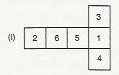#### Question 4:

Draw nets for each of the following polyhedrons:(i)(ii)(iii)(iv)#### Question 5:

Match the following figures: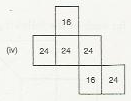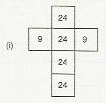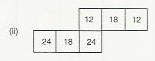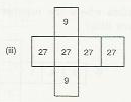#### Question 1:

What is the least number of planes that can enclose a solid? What is the name of the solid?

#### Question 2:

Can a polyhedron have for its faces:
(i) 3 triangles?
(ii) 4 triangles?
(iii) a square and four triangles?

#### Question 3:

Is it possible to have a polyhedron with any given number of faces?

#### Question 4:

Is a square prism same as a cube?

#### Question 5:

Can a polyhedron have 10 faces, 20 edges and 15 vertices?

#### Question 6:

Verify Euler's formula for each of the following polyhedrons:View NCERT Solutions for all chapters of Class 8